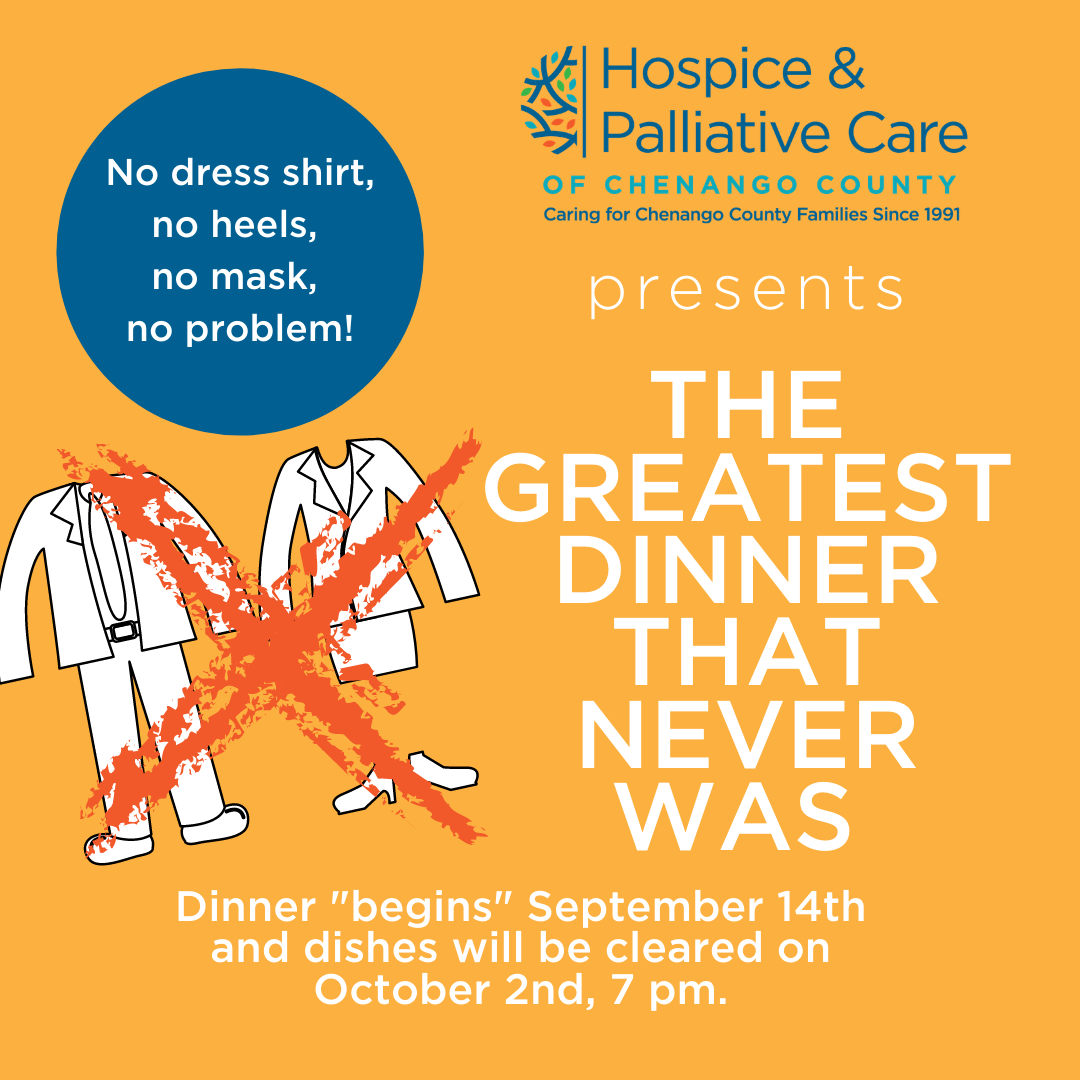See our COVID-19 update >## Greatest Dinner Event!!

Time left to register!

## jQuery(document).ready(function(){ // Set the date we're counting down to var countDownDate = new Date("Oct 1, 2020 23:59:59").getTime(); // Update the count down every 1 second var x = setInterval(function() { // Get today's date and time var now = new Date().getTime(); // Find the distance between now and the count down date var distance = countDownDate - now; // Time calculations for days, hours, minutes and seconds var days = Math.floor(distance / (1000 * 60 * 60 * 24)); var hours = Math.floor((distance % (1000 * 60 * 60 * 24)) / (1000 * 60 * 60)); var minutes = Math.floor((distance % (1000 * 60 * 60)) / (1000 * 60)); var seconds = Math.floor((distance % (1000 * 60)) / 1000); // Output the result in an element with timer_id document.getElementById("timer-Oct-1--2020-23-59-59").innerHTML = days + "d " + hours + "h " + minutes + "m " + seconds + "s "; // If the count down is over, write some text if (distance < 0) { clearInterval(x); document.getElementById("timer-Oct-1--2020-23-59-59").innerHTML = "EXPIRED"; } }, 1000); });

### Since 1991